##### ACT For Dummies: Book + 3 Practice Tests Online + Flashcards, 7th Edition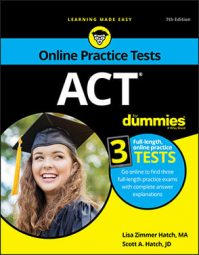Many of the geometry problems on the ACT require you to know a lot about triangles. Remember the facts and rules about triangles given here, and you’re on your way to acing geometry questions.

## Classifying triangles

Triangles are classified based on the measurements of their sides and angles. Here are the types of triangles you may need to know for the ACT:
• Equilateral: A triangle with three equal sides and three equal angles.
• Isosceles: A triangle with two equal sides and two equal angles. The angles opposite equal sides in an isosceles triangle are also equal.
• Scalene: A triangle with no equal sides and no equal angles.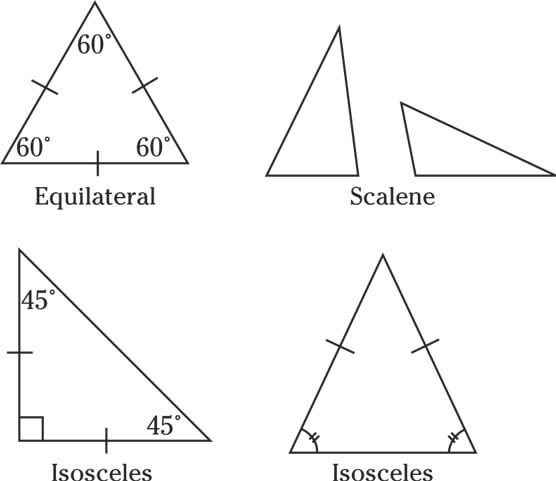Equilateral, isosceles, and scalene triangles.

## Sizing up triangles

When you’re figuring out ACT questions that deal with triangles, you need to know these rules about the measurements of their sides and angles:
• In any triangle, the largest angle is opposite the longest side.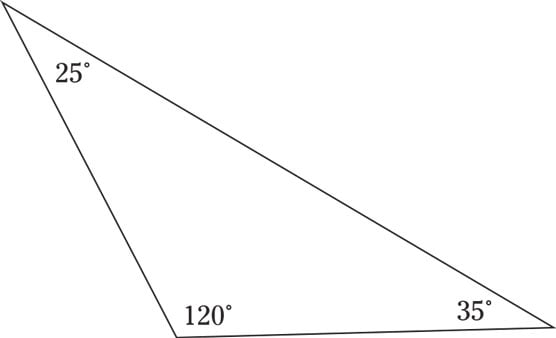The largest angle is opposite the longest side.
• In any triangle, the sum of the lengths of two sides must be greater than the length of the third side.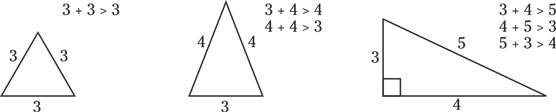The sum of the lengths of two sides of a triangle is greater than the length of the third side.
• In any type of triangle, the sum of the interior angles is 180 degrees.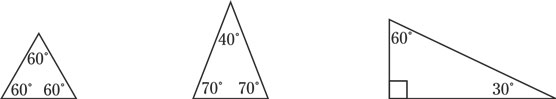The sum of the interior angles of a triangle is 180 degrees.
• The measure of an exterior angle of a triangle is equal to the sum of the two remote interior angles.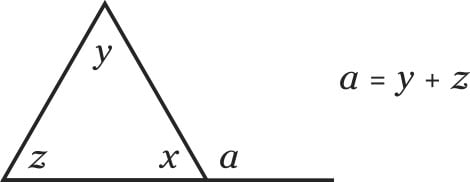The measure of an exterior angle is equal to the sum of the two remote interior angles.

## Zeroing in on similar triangles

Several ACT math questions require you to compare similar triangles. Similar triangles look alike but are different sizes. Here’s what you need to know about similar triangles:
• Similar triangles have the same angle measures. If you can determine that two triangles contain angles that measure the same degrees, you know the triangles are similar.
• The sides of similar triangles are in proportion. For example, if the heights of two similar triangles are in a ratio of 2:3, then the bases of those triangles are also in a ratio of 2:3.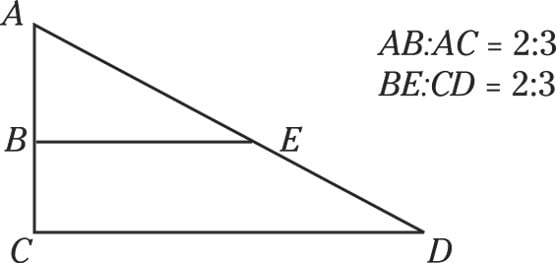Similar triangles have proportionate sides.

Don’t assume that triangles are similar on the ACT just because they look similar to you. The only way you know two triangles are similar is if the test tells you they are or you can determine that their angle measures are the same.